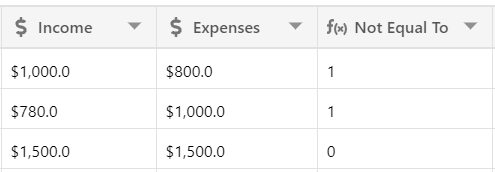Logical Operations in a programming-language symbol that denotes a logical operation. In stackby we use logical operators that allow you to compare values in one column to another column.

Greater than or less than

We will have two columns income and expenses, from that we will find whether it is greater than or less that with the help of operator in Stackby

Greater than : If income is greater than expenses, we will check this. The result will be either true(1) or false(0) as.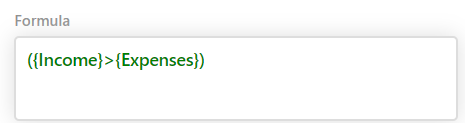Result: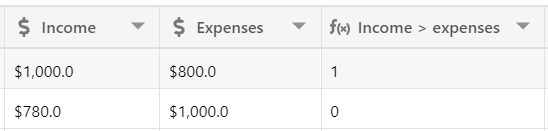Less than : If income is less than expenses, we will check. The result will be either true(1) or false(0).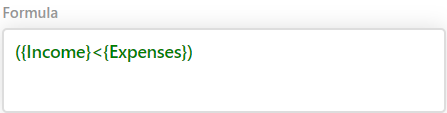Result: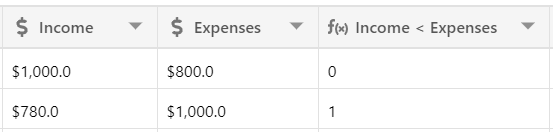Greater  than or equal to or less than or equal to

Greater than or equal to

We have seen that if income is greater than expenses the result will be 0 or 1. But what if both are the same?  So If income is greater than equal to expenses then the result will be 1(true).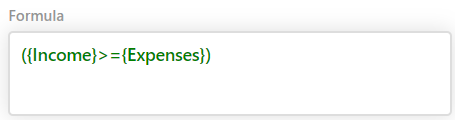Result: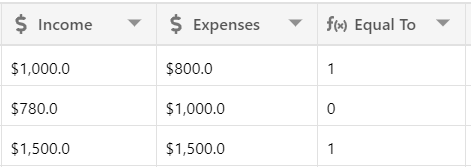Less than or equal to

In the same way, If income is less than equal to expenses then the result will be 1(true).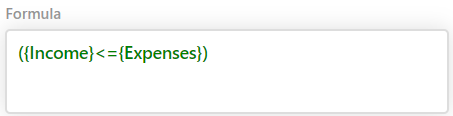Result: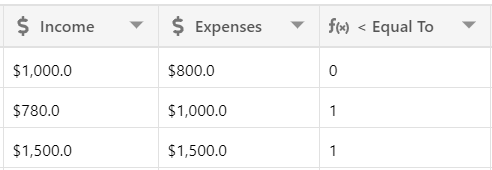Equal : Check if income is exactly equal to expenses. We will use “=” operator.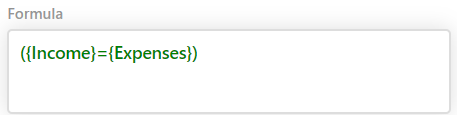Result: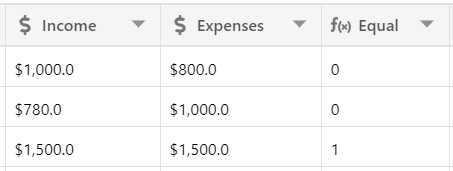Does not equal: Same, we can check if a value is not equal to another by using “!=” operator.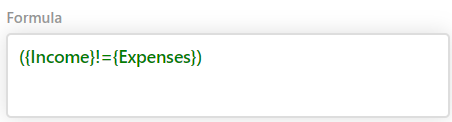Result: